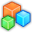# Microsoft Small BasicProgram Listing: ZKQ163
xsegs = 32
ysegs = 32

far = 1024
near = 0.1

'360 degrees
pi2 = Math.Pi * 2

'Field of view
f = 1.0 / Math.Tan(Math.Pi / 4)

' Calculate total number of segments to draw
total = xsegs * ysegs / 2

'Specifies if line drawing has already started
started = 0

'Old coordinates for line drawing (from)
oldx = 0
oldy = 0

'Temporary variables for calculating x, y and z
fx = 0
fy = 0
fz = 0

' Draw vertical rings
For i = 0 To total + xsegs
' Calculate current Y segment
yseg = Math.Remainder(i, xsegs)
' Calculate curretn Y-axis slice
xseg = Math.Floor(i / xsegs)

' Calculate angle for current vertex
angle_x = pi2 * (xseg / (xsegs - 1))
angle_y = pi2 * (yseg / (ysegs - 1))

'Calculate 3D vertex
Calc3D()
' Draw 3D line
DrawLine()
' Stop drawing when we have reached the end of the slice
If(yseg = ysegs) Then
started = 0
EndIf
EndFor

started = 0
' Draw horizontal rings
For i = 0 To total + ysegs
' Calculate current X segment
xseg = Math.Remainder(i, ysegs)
' Calculate current X-axis slice
yseg = Math.Floor(i / ysegs)

'Calculate angle
angle_x = pi2 * (xseg / (xsegs - 1))
angle_y = pi2 * (yseg / (ysegs - 1))

'Calculate 3D vertex
Calc3D()
'Draw 3D line
DrawLine()
'End drawing when we have reached the end of the slice
If(xseg = xsegs - 1) Then
started = 0
EndIf
EndFor

Sub Calc3D
fx = (Math.Sin( angle_x ) * Math.Sin( angle_y )) * radius
fy = (Math.Cos( angle_y )) * radius
fz = (Math.Cos( angle_x ) * Math.Sin( angle_y )) * radius + 4
EndSub

'Draws a 3D line using x, y and z variables
Sub DrawLine
'Calculate 3D projection
x = fx * f
y = fy * f
z = fz * ((far + near)/(far-near)) + 1.0
w = (2*near*far)/(near-far)
If w <> 0 Then
x = x / z * w
y = y / z * w
z = z / z * w

' Move 2D screen coordinate to center for screen
x = (x * GraphicsWindow.Width) / (2.0 * w) + (GraphicsWindow.Width / 2)
y = (y * GraphicsWindow.Height) / (2.0 * w) + (GraphicsWindow.Height / 2)

'Only draw line, if we have at least calculated one earlier vertex
If started <> 0 Then
GraphicsWindow.DrawLine(oldx, oldy, x, y)
Endif

' Drawing started
started = 1

'Kepp old vertex position for next line
oldx = x
oldy = y
EndIf
EndSub